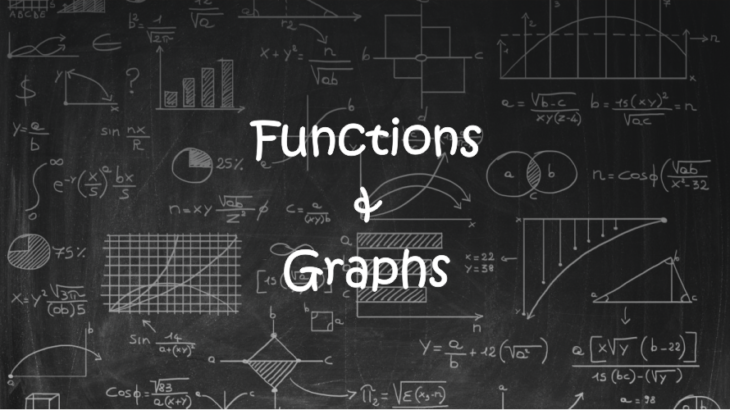# Basic Concepts of Functions and Graphs for CAT Exams

Basic Concepts of Functions and Graphs for CAT Exams
4.5 (90.81%) 37 vote[s]

In CAT Exam, certain kind of questions from algebra requires higher levels of algebraic concepts. However, such questions can also be easily solved by using alternate approaches from graphs.

Below are a few questions from CAT and other exams, which would be very difficult to crack if we try solving them using the concepts from algebra.

1. Find the number of real solutions of x for the equation ${2^x} = {x^2}$.
2. Find the number of integral solution the equation |x-1| + |x+3| +|x-5| <20
3. How many integral pairs (x, y) are there such that |x|+|y|=4
4. Find the minimum value of y if y=|1-|x+3||

As I mentioned in the introduction, all of the above four questions can be solved by using the concept of algebra.  But if we have the basic idea of the graphs of some special functions and a good understanding of the transformation of the graphs, then we can solve complex looking questions easily.

In this article, we will be focusing on the concepts of graphs and the process of transformation of the graphs. In the next article, we will understand how to use these concepts to solve the types of questions given above.Let us understand some special functions which appear very frequently.

### Identity function/ graph of f(x)=x:

A function f defined by f(x) = x for all real value of x, is called the identity function.

If you refer to the graph, you will find that it is nothing but a straight line passing through the origin and inclined an angle of 45° with the x-axis.  Algebraically, we can also represent it as y=x.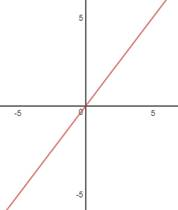### Graph of $f(x) = {x^2}$

A function given by $f(x) = {x^2}$  is called the square function.

Clearly, $y = {x^2}$  is a parabola and it’s also an even function,  so it is symmetrical about y-axis as shown in the figure: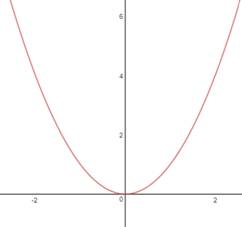### Graph of $f(x) = {x^3}$

A function given by $f(x) = {x^3}$  is called the cube function. When a function is an odd function, its graph is symmetrical about the opposite quadrant or we can say that the graph is symmetrical about the origin as shown in the figure: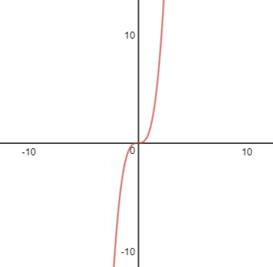On analyzing and extending the logic of the above three graph, we can generalize the following:

#### Graph of $f(x) = {x^{2n}}$;  Where n is a natural number.

This will always be an even function.  Hence, the graph of $f(x) = {x^{2n}}$  will always be symmetrical about the y-axis.

The below figure shows graphs of the function for some values of n.Graph of $f(x) = {x^{2n – 1}}$;  Where n is a natural number.

This will always be an odd function.  Hence, the graph of $f(x) = {x^{2n – 1}}$  will always be symmetrical about the origin or opposite quadrants.

The below figure shows graphs of the function for some values of n.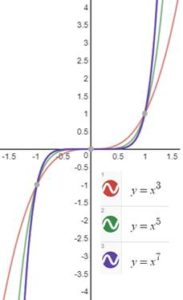Graphs of rational expressions

Rational functions are the functions obtained by dividing a polynomial by another polynomial, i.e. $f(x) = \frac{{P(x)}}{{Q(x)}}$

In the CAT exam, we don’t get complicated rational expressions. Rather, we get simple rational expression functions, and their graphs are easy to plot as well.

Let us take some elementary rational functions and their corresponding graphs.

1. Graph of $f(x) = \frac{1}{x}$

This is also called the reciprocal function or a rectangular hyperbola. This is also an odd function so its graph is symmetrical about the opposite quadrant.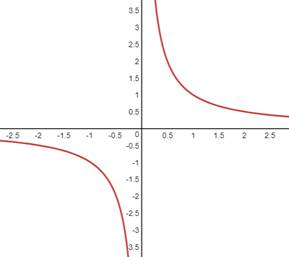1. Graph of $f(x) = \frac{1}{{{x^2}}}$

Since the power of x is even, clearly, this is an even function. So it must be symmetrical about the y-axis. Also, even negative values of the function f(x) will always be positive.  It is also important to mention here that the domain of the function will be all real values except 0.  that is, it cannot take the value 0.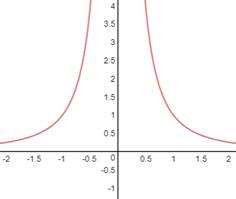## Piecewise functions

There are a few types of functions which come under the category of piecewise functions. They are absolute value function, signum function, greatest integer function, fractional part function, least integral function etc.

For CAT exams, we have to focus only on the absolute value function in detail.

### Absolute value function (or modulus function):

• $y=|x|=\left\{ \begin{array}{*{35}{l}} x; & x\ge 0 \\ x;\text{ }\!\!~\!\!\text{ } & x<0 \\\end{array} \right.$ .It is a numerical value of x
• It is symmetrical about y axis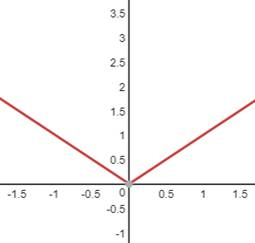Properties of modulus functions:

• $|x| \le a \Rightarrow – a \le x \le a;(a \ge 0)$
• $|x| \ge a \Rightarrow x \le – a\quad {\rm{ or }}x \ge a;(a \ge 0)$
• $|x \pm y| \ge ||x| – |y||$

### Exponential function

The function $f(x) = {a^x}$, where $a \ne 0$, and x  is a real number,  is called exponential function.

Depending upon the value of a, we can have all the increasing function or decreasing function.

Case I: If a >1

Say, $f(x) = y = {4^x}$  increases with the increase in x.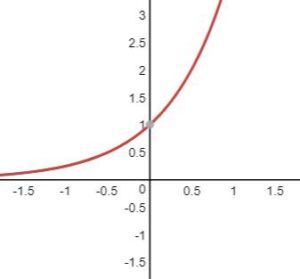Case II: If 0< a < 1

Say, $f(x) = y = {\left( {\frac{1}{2}} \right)^x}$  decreases with an increase in x.

In general, exponential function increases or decreases as (a>1) or (0<a<1) respectively.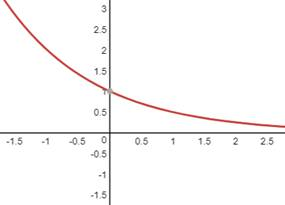### Logarithmic function

This is an inverse of an exponential function. In other words, in the exponential function if we replace x with y and y with x, and rearrange the function taking x as the independent variable and as the dependent variable, then the new function will be a logarithmic function.

The logarithmic function is defined as $f\left( x \right) = {\log _a}x;\;(x,\;a > 0)$ and $a \ne 0$. The domain of logarithmic function is all positive real number.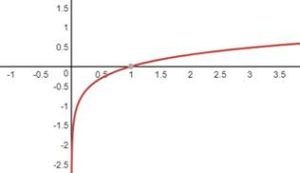These are the graphs of basic functions which frequently appeared in the CAT exam. Our next step is to understand the transformation of graphs.

## Transformation of the Graphs

### Transformation of function f(x) to $f(x) \pm a$

When f(x) is transformed to f(x)+a where a is positive, the graph shifts upward through ‘a’ units.

And if f(x) is transformed to f(x)+a where a is negative, the graph shifts downward through ‘a’ units.

Let us understand it with the help of an example:

Say, where to plot the graph of y=|x|+3.

We know that y=|x| is plotted as below: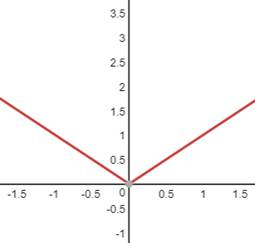So, fo y=|x|+3, the graph will shift 2 units upward, i.e,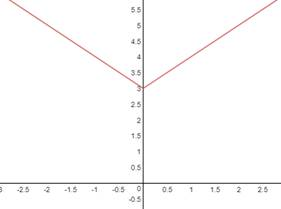Similarly, if we have to plot y=|x| -2, the graph will shift downwards by 2 units.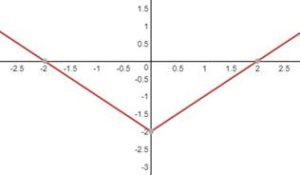### Transformation of graph from f(x) to $f(x \pm a)$

For f(x) à f(x+a), where a is positive, the graph shifts through ‘a’ units towards the left. And for f(x) à f(x-a), the graph shifts through ‘a’ units towards the right.

Example: Plot the graph of y=|x+3| and y=|x-2|

The graph of y=|x+3| will be: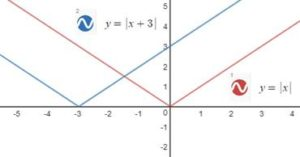Similarly, the graph of y=|x-2| will be: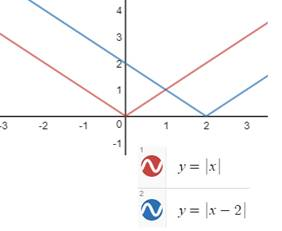### Transformation of graph from f(x) to f(-x)

To draw y=f(-x), take the image of the curve y=f(x) in y-axis as a plane mirror. Or, turn the graph of f(x) by 1800 about the y-axis.

Example: Plot the graph of $y = {4^{ – x}}$, where a is positive.

We know the graph of $y = {4^x}$

Taking the mirror image of the graph around the y-axis.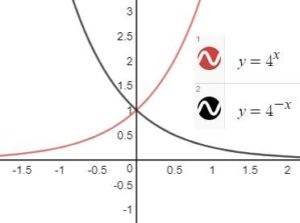### Transformation of graph from f(x) to -f(x)

To draw y=-f(x) take image of y=f(x) in the x-axis as plane mirror. Or, turn the graph of f(x) by 1800 about x-axis.

Example: Plot the graph of y=-4x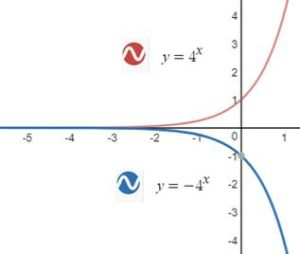Example: Plot the graph of y=-|x|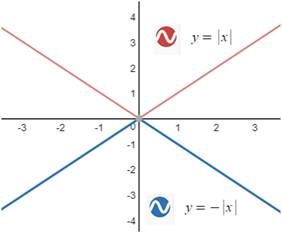### Transformation of graph from f(x) to -f(-x)

To draw y=-f(-x) take image of f(x) about y-axis to obtain f(-x) and then take image of f(-x) about x-axis to obtain –f(-x).

Example: Plot the graph of y=-4-x

Taking mirror image about the y-axis to get the graph of y=4-x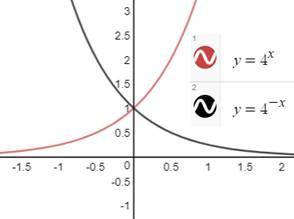Taking mirror image of y=4-x about x-axis to get the y=-4-x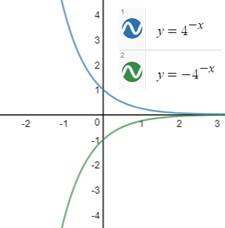### Transformation of graph from f(x) to |f(x)|

The graph of |f(x)| is drawn by taking the mirror image (in x-axis) of the portion of the graph of f(x) which lies below x-axis.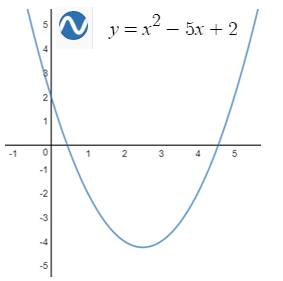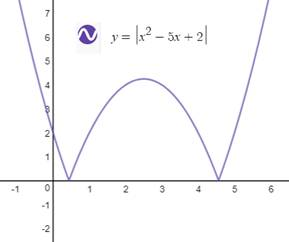### Transformation of graph from f(x) to f(|x|)

To draw the graph of y=f(|x|), neglect the curve for x<0 and take the images of curve for x≥ 0 about the y-axis.

Example: Plot the graph of y=|x|2-5|x|+2

We know the graph of y=x2-5x+2Neglecting graph for x<0 and Taking mirror image about y-axis to the part of the graph for x≥0.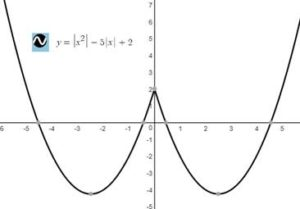There are a few more concepts on transformation, but the concepts used above are sufficient to deal with questions which appear in CAT exam.